Year 8 Interactive Maths - Second Edition

## Circles and using a Compass

In this section, we will consider the definition of a circle, lines in a circle and parts of a circle.

### Circle

A circle is a set of points in a plane that are the same distance from a fixed point (called the centre). These set of points form the perimeter of the circle.

The radius is the distance from the centre of the circle to any point on its perimeter.

The circumference of a circle is the perimeter of the circle.

These parts of a circle are indicated in the accompanying diagram.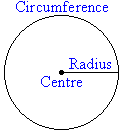### Lines in a Circle

The name of a line in a circle depends on its position in the circle.

A secant is a line that passes through any two points on a circle.

A chord is a line that joins two points on the circumference of a circle.

The diameter is a chord that passes through the centre of a circle.

A tangent is a line that touches the circle at only one point.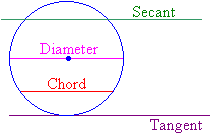### Parts of a Circle

An arc is a part of the circumference.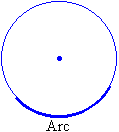A sector is the part of a circle between two radii.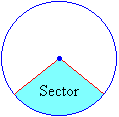A segment is the part of a circle that is between a chord and the circumference.A semicircle is a half of a circle.### Compass

A compass is an instrument used to draw circles or the parts of circles called arcs.  It consists of two movable arms hinged together where one arm has a pointed end and the other arm holds a pencil.

Note that a compass is also called a pair of compasses.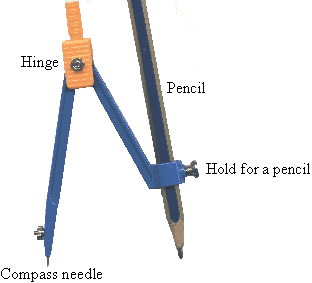To draw a circle (or arc) with a compass:

• make sure that the hinge at the top of the compass is tightened so that it does not slip
• tighten the hold for the pencil so it also does not slip
• align the pencil lead with the compass's needle
• press down the needle and turn the knob at the top of the compass to draw a circle (or arc)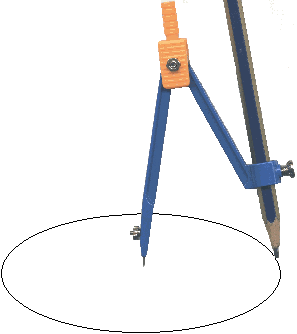#### Example 2

Use a compass to draw a circle of radius 4 cm.

##### Solution:

Step 1:  Use a ruler to set the distance from the point of the compass to the pencil's lead at 4 cm.
Step 2:  Place the point of the compass at the centre of the circle.
Step 3:  Draw the circle by turning the compass through 360º.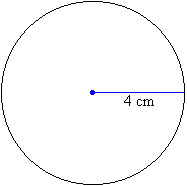### Activity 10.1

1.  Use a compass to draw a circle of radius 5 cm.
2.  Use a compass to draw a circle of diameter 12 cm.

3a.  Use a compass to draw a circle of radius 4.5 cm.
3b.  Draw the diameter of the circle; and use a ruler to measure the length of the diameter.
3c.  Write an equation to represent the relation between the radius, r, and the diameter, d.

4a.  Use a compass to draw a circle of radius 5.5 cm.
4b.  Draw a diameter and label it PQ.
4c.  Draw a triangle PQR where R is on the semicircle.
4d.  Use a protractor to measure the size of angle PRQ.

5a.  Use a compass to draw a circle of radius 6.5 cm.
5b.  Draw a diameter and label it PQ.
5c.  Draw a triangle PQR where R is on the semicircle.
5d.  Use a protractor to measure the size of angle PRQ.

6a.  Use a compass to draw a circle of radius 7.5 cm.
6b.  Draw a diameter and label it PQ.
6c.  Draw a triangle PQR where R is on the semicircle.
6d.  Use a protractor to measure the size of angle PRQ.

7.  Use the results of questions 4, 5 and 6 to complete the following statements:
a.  The size of the angle on the diameter of a circle with a vertex on the circle is …
b.  If a triangle is drawn in a semicircle using the diameter as an edge, the angle touching the curved part of the triangle is …